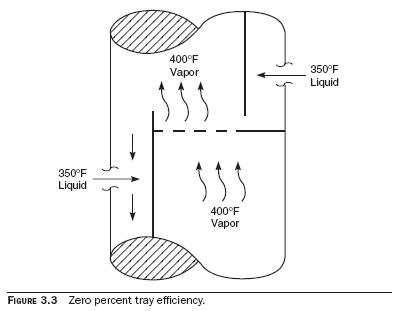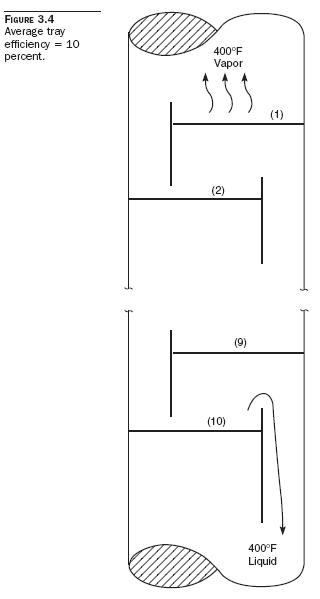﻿ How to Calculate Tray Efficiency | Oil & Gas Process Engineering

## How to Calculate Tray Efficiency

Distillation trays in a fractionator operate between 10 and 90 percent efficiency. It is the process person’s job to make them operate as close to 90 percent efficiency as possible. Calculating tray efficiency is sometimes simple. Compare the vapor temperature leaving a tray to the liquid temperature leaving the trays. For example, the efficiency of the tray shown in Fig. 3.2 is 100 percent. The efficiency of the tray in Fig. 3.3 is 0 percent.How about the 10 trays shown in Fig. 3.4? Calculate their average efficiency (answer is 10 percent). As the vapor temperature rising from the top tray equals the liquid temperature draining from the bottom tray, the 10 trays are behaving as a single perfect tray with 100 percent efficiency. But as there are 10 trays, each tray, on average, acts like one-tenth of a perfect tray.

Poor tray efficiency is caused by one of two factors:
• Flooding
• Dumping